# Toomre Examples¶

This project is intended for observing the behavior of stars in close galactic interactions. To approximate a close encounter, one galaxy was placed at the origin of a reference frame, while the other was placed on a perfect parabolic trajectory around the "stationary" galaxy. The stationary galaxy is a point mass with 120 massless stars orbitting around it, while the disrupting galaxy is simply a point mass. The stars are placed in specific rings around the center mass to create an even distribution of particles for clear observation. Four different examples were used to observe the movement of stars under various situations. The first example is called a Direct Passage. The disruptor galaxy has the same mass as the stationary galaxy, and it approaches the stationary galaxy with the same direction as the stars are orbitting. The second example is called a Retrograde Passage. The disruptor galaxy has the same mass as the stationary galaxy, but it approaches with an opposite direction in relation to the stars' orbit. The third case is called a Light Mass Disruptor. It is the same as the direct passage case, except that the approaching galaxy has a quarter of the mass of the stationary galaxy. The fourth case is called the Heavy Mass Disruptor. In this situation the passage is direct just as with the light mass disruptor, but the approaching galaxy has four times the mass of the stationary galaxy.

In :
%pylab inline
import numpy as np
import matplotlib.pyplot as plt
from scipy.integrate import odeint
from IPython.html.widgets import interact, interactive, fixed

Populating the interactive namespace from numpy and matplotlib

C:\Users\masha\Anaconda3\lib\site-packages\IPython\html.py:14: ShimWarning: The IPython.html package has been deprecated. You should import from notebook instead. IPython.html.widgets has moved to ipywidgets.
"IPython.html.widgets has moved to ipywidgets.", ShimWarning)

In :
import ToomreGalaxy as ToomreGalaxy

In :
#ToomreGalaxy.Generate_Data()


## Viewing Results¶

Instructions:

Four interactive plots that represent the four fundamental Toomre cases are imported below. In order to create the interactive plot, the user must simply run the single code cell associated with each case by holding the shift key and pressing the enter key on their keyboard. Then, the user can slide the time bar to see how the galactic encounters affect the movement of the stars over time.

#### Case 1: Direct Passage¶

In :
a = interact(ToomreGalaxy.Make_Plot_stars,results = fixed(np.load('Toomre_A.npy')), M = fixed(1.0e11), S = fixed(1.0e11), t = (0.1,20.1), dt = fixed(0.0075))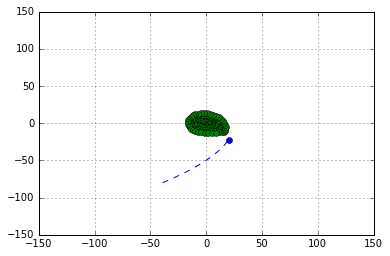#### Case 2: Retrograde Passage¶

In :
b = interact(ToomreGalaxy.Make_Plot_stars,results = fixed(np.load('Toomre_B.npy')), M = fixed(1.0e11), S = fixed(1.0e11), t = (0.1,20.1), dt = fixed(0.0075))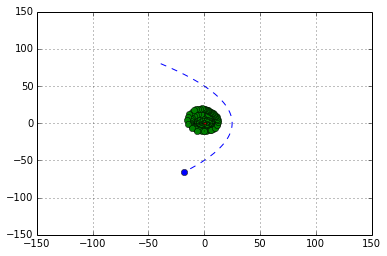#### Case 3: Light Mass Disruptor¶

In :
c = interact(ToomreGalaxy.Make_Plot_stars,results = fixed(np.load('Toomre_C.npy')), M = fixed(1.0e11), S = fixed(1.0e11/4), t = (0.1,20.1), dt = fixed(0.0075))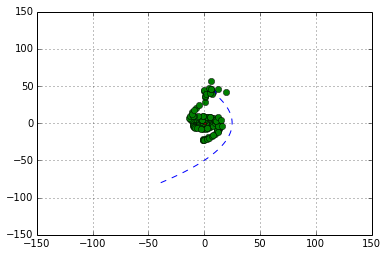#### Case 4: Heavy Mass Disruptor¶

In :
d = interact(ToomreGalaxy.Make_Plot_stars,results = fixed(np.load('Toomre_D.npy')), M = fixed(1.0e11), S = fixed(1.0e11*4), t = (0.1,20.1), dt = fixed(0.0075))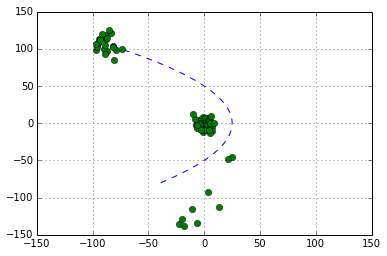## Interactive Viewing Results¶

Instructions:

There are two code cells for each of the four Toomre cases below. The first cell imports the function that calculates the positions of all of the stars and the disruptor galaxy for every timestep. In this cell it is possible to experiment with the function's parameters in order to see how various factors affect the movement of the stars.

When all of the parameters are set, the user must then hold the shift key while pressing the enter key to the run the cell. Both cells for each case must be run in consecutive order to produce interactive plots.

Once the plots are made, it is possible to move the time slider left and right. The time by default ranges from 0 to 20, which is equivilant to a range of 20 * 10^8 years. Moving the slider from left to right allows the user to see the consecutive progression of the star disruptions.

Parameter Key:

• Case:

• 1 Corresponds with direct passage case
• 2 Corresponds with retrograde passage case
• 3 Corresponds with light mass disruptor case
• 4 Corresponds with heavy mass disruptor case
• The purpose of this parameter is to set the positions of the rings of stars. For the first three cases, the stars are placed in rings that are 20%, 30%, 40%, 50%, and 60% of the Rmin value which is the minimum distance between the disruptor galaxy and the stars. But to produce the best results for the 4th case, the rings must be placed at 12%, 18%, 24%, 30%, and 36% of the Rmin distance. When adjusting the parameters, the user must choose the case that is most similar to their experiment to produce the best results.
• Rx0: x component of the initial position of the disruptor galaxy

• Ry0: y component of the initial position of the disruptor galaxy

• Initial_Velocity_X: x component of the initial velocity of the disruptor galaxy

• Initial_Velocity_Y: y component of the initial velocity of the disruptor galaxy

• t: Maximum time to which the function calculates the star and disruptor galaxy positions. A suggested experiment would be to increase the time to see what happens to the stars long after the disruptor galaxy passes.

• M: Mass of the "stationary" galaxy

• S: Mass of the disruptor galaxy

• dt: time steps that the function uses when integrating differential equations that describe the motion of the stars and disruptor galaxy. Decreasing dt will prolong the time it takes to run the function, but will produce more accurate results.

#### Case 1: Direct Passage¶

In :
results_1 = ToomreGalaxy.Make_Master_Array(Case = 1, Rx0 = -39., Ry0 = -80., M = 1.0e11, S = 1.0e11)

In :
a1 = interact(ToomreGalaxy.Make_Plot_stars,results = fixed(results_1), M = fixed(1.0e11), S = fixed(1.0e11), t = (0.1,20.1), dt = fixed(0.0075))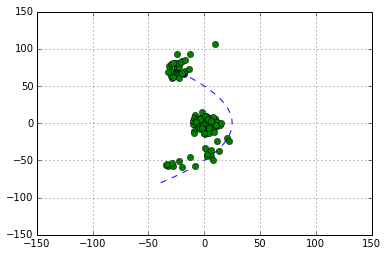#### Case 2: Retrograde Passage¶

In :
results_2 = ToomreGalaxy.Make_Master_Array(Case = 2, Rx0 = -39., Ry0 = 80., M = 1.0e11, S = 1.0e11)

In :
b1 = interact(ToomreGalaxy.Make_Plot_stars,results = fixed(results_2), M = fixed(1.0e11), S = fixed(1.0e11), t = (0.1,20.1), dt = fixed(0.0075))#### Case 3: Light Mass Disruptor¶

In :
results_3 = ToomreGalaxy.Make_Master_Array(Case = 3, Rx0 = -39., Ry0 = -80., M = 1.0e11, S = 1.0e11/4)

In :
c1 = interact(ToomreGalaxy.Make_Plot_stars,results = fixed(results_3), M = fixed(1.0e11), S = fixed(1.0e11/4), t = (0.1,20.1), dt = fixed(0.0075))##### Case 4: Heavy Mass Disruptor¶
In :
results_4 = ToomreGalaxy.Make_Master_Array(Case = 4, Rx0 = -39., Ry0 = 80., M = 1.0e11, S = 1.0e11*4)

In :
d1 = interact(ToomreGalaxy.Make_Plot_stars,results = fixed(results_4), M = fixed(1.0e11), S = fixed(1.0e11*4), t = (0.1,20.1), dt = fixed(0.0075))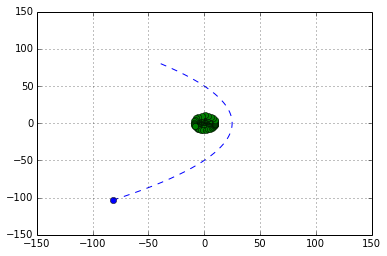## Interactive Viewing Results - Highlighted Star¶

Instructions:

There are two code cells for each of the four Toomre cases below. The first cell imports the function that calculates the positions of all of the stars and the disruptor galaxy for every timestep. This function also highlights one star by coloring it green. In this cell it is possible to experiment with the function's parameters in order to see how various factors affect the movement of the stars. The primary benefit of having a highlighted star, is to easily be able to visually follow its movement over time.

When all of the parameters are set, the user must then hold the shift key while pressing the enter key to the run the cell. Both cells for each case must be run in consecutive order to produce interactive plots.

Once the plots are made, it is possible to move the two sliders left and right to change the time and green star index. The time by default ranges from 0 to 20, which is equivilant to a range of 20 * 10^8 years. The star index ranges from 1 to 120, and scrolling the widget bar changes this index. The first star is located in the inner most ring at 0 pi radians, and the index increases along the unit circle. When the index moves through all of the stars in the full 2 pi radian circle, it jumps to the adjacent ring that is surrounding it to the 0 pi radian position.

Parameter Key:

• Case:

• 1 Corresponds with direct passage case
• 2 Corresponds with retrograde passage case
• 3 Corresponds with light mass disruptor case
• 4 Corresponds with heavy mass disruptor case
• The purpose of this parameter is to set the positions of the rings of stars. For the first three cases, the stars are placed in rings that are 20%, 30%, 40%, 50%, and 60% of the Rmin value which is the minimum distance between the disruptor galaxy and the stars. But to produce the best results for the 4th case, the rings must be placed at 12%, 18%, 24%, 30%, and 36% of the Rmin distance. When adjusting the parameters, the user must choose the case that is most similar to their experiment to produce the best results.
• Rx0: x component of the initial position of the disruptor galaxy

• Ry0: y component of the initial position of the disruptor galaxy

• Initial_Velocity_X: x component of the initial velocity of the disruptor galaxy

• Initial_Velocity_Y: y component of the initial velocity of the disruptor galaxy

• t: Maximum time to which the function calculates the star and disruptor galaxy positions. A suggested experiment would be to increase the time to see what happens to the stars long after the disruptor galaxy passes.

• M: Mass of the "stationary" galaxy

• S: Mass of the disruptor galaxy

• dt: time steps that the function uses when integrating differential equations that describe the motion of the stars and disruptor galaxy. Decreasing dt will prolong the time it takes to run the function, but will produce more accurate results.

#### Case 1: Direct Passage¶

In :
results_A = ToomreGalaxy.Make_Master_Array(Case = 1, Rx0 = -39., Ry0 = -80., M = 1.0e11, S = 1.0e11)

In :
A = interact(ToomreGalaxy.Make_Plots_Yellow_Star,results = fixed(results_A), M = fixed(1.0e11), S = fixed(1.0e11*4), t = (0.1,20.1), dt = fixed(0.0075), YellowStar = (1,120))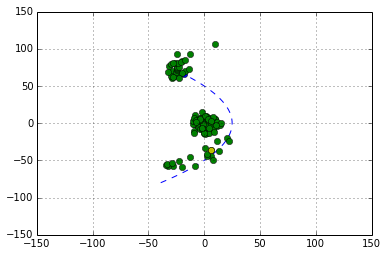#### Case 2: Retrograde Passage¶

In :
results_B = ToomreGalaxy.Make_Master_Array(Case = 2, Rx0 = -39., Ry0 = 80., M = 1.0e11, S = 1.0e11)

In :
B = interact(ToomreGalaxy.Make_Plots_Yellow_Star,results = fixed(results_B), M = fixed(1.0e11), S = fixed(1.0e11), t = (0.1,20.1), dt = fixed(0.0075), YellowStar = (1,120))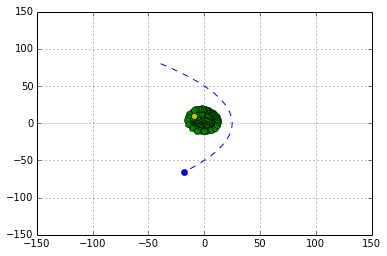#### Case 3: Light Mass Disruptor¶

In :
results_C = ToomreGalaxy.Make_Master_Array(Case = 3, Rx0 = -39., Ry0 = -80., M = 1.0e11, S = 1.0e11/4)

In :
C = interact(ToomreGalaxy.Make_Plots_Yellow_Star,results = fixed(results_C), M = fixed(1.0e11), S = fixed(1.0e11/4), t = (0.1,20.1), dt = fixed(0.0075), YellowStar = (1,120))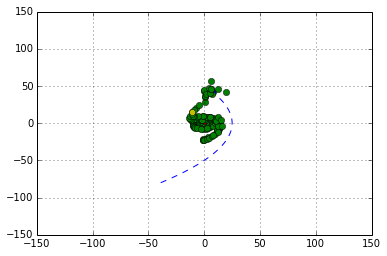#### Case 4: Heavy Mass Disruptor¶

In :
results_D = ToomreGalaxy.Make_Master_Array(Case = 4, Rx0 = -39., Ry0 = -80., M = 1.0e11, S = 1.0e11*4)

In :
D = interact(ToomreGalaxy.Make_Plots_Yellow_Star,results = fixed(results_D), M = fixed(1.0e11), S = fixed(1.0e11*4), t = (0.1,20.1), dt = fixed(0.0075), YellowStar = (1,120))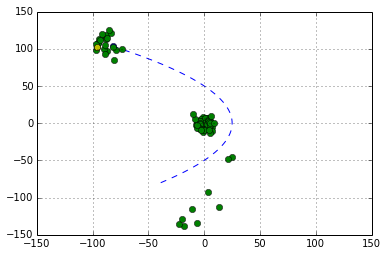In :